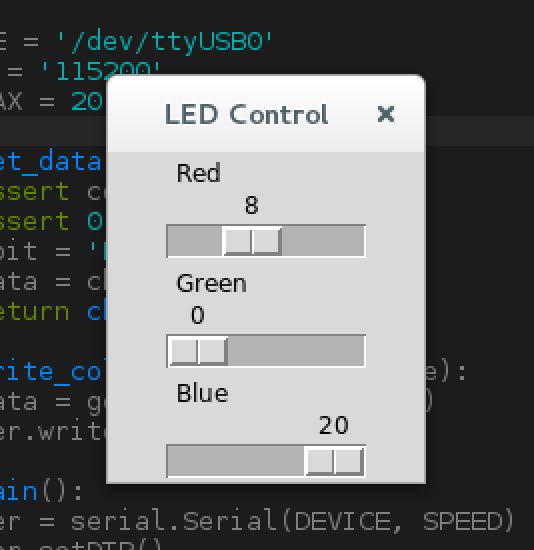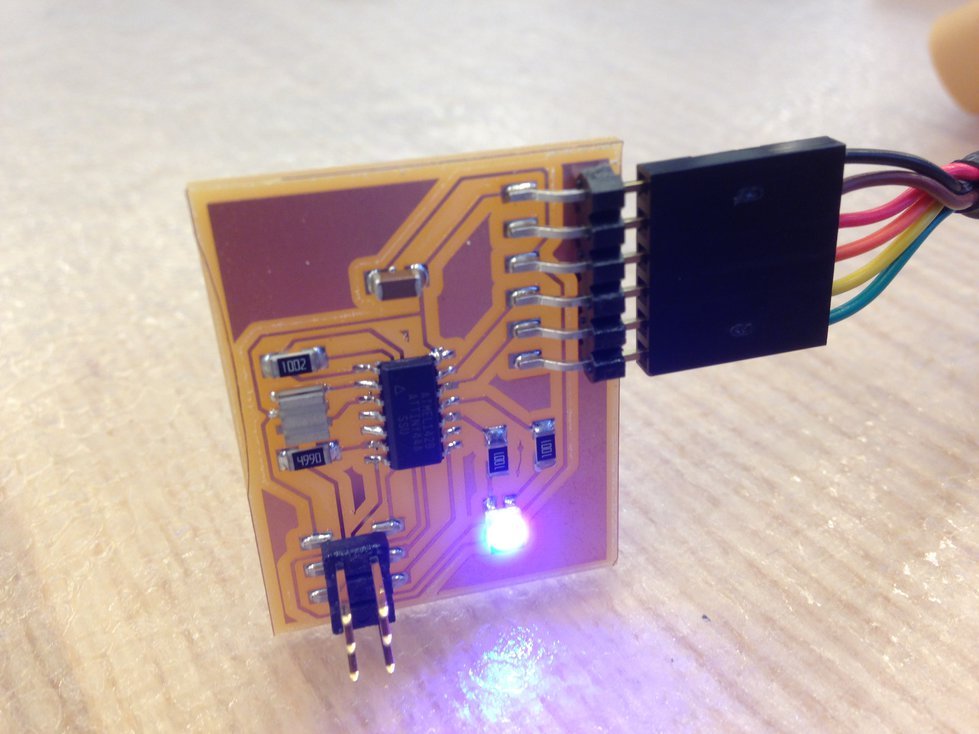# Week 12: Interface and Application Programming

The assignment for this week was to write an application that interfaces with an input or output device that we made. For this week, I decided to write a GUI to control my RGB LED.

# Embedded Code

First, I had to write embedded code that could let me control the RGB LED in an interesting way. I wrote a clock interrupt-based PWM routine that let me independently set the R, G, and B channels to one of 20 brightness values. Next, I wrote code to take commands over serial to change the LED values. I used a simple protocol, where a control message was contained in a single byte. The upper 2 bits were used to set the color, and the lower 6 bits were used to set the intensity. Decoding was as simple as:

color = input >> 6
value = input & 0x3f


There were some challenges with getting the serial input to work correctly. After some debugging, I found that I needed to disable interrupts while reading a byte in from serial (otherwise, the timing would get messed up by the LED PWM).

My final code for my ATtiny44 is available here: https://gist.github.com/a2e7e2bb7718dc1c2a4ddbdcc317898a.

# GUI Code

Before writing the GUI, I wrote a simple test program to verify that communication over serial worked as expected.

After that, I used the TkInter library to write a simple GUI in Python:

import serial
import time
from Tkinter import *

DEVICE = '/dev/ttyUSB0'
SPEED = '115200'
PWM_MAX = 20

def get_data(color, value):
assert color in 'RGB'
assert 0 <= value <= PWM_MAX
cbit = 'RGB'.index(color)
data = cbit << 6 | value
return chr(data)

def write_color(ser, color, value):
data = get_data(color, value)
ser.write(data)

def main():
ser = serial.Serial(DEVICE, SPEED)
ser.setDTR()
ser.flushInput()
ser.flushOutput()

master = Tk()
master.wm_title('LED Control')
def set_color(color):
def setter(value):
value = int(value)
write_color(ser, color, value)
return setter
scale = Scale(
master,
from_=0,
to=PWM_MAX,
orient=HORIZONTAL,
command=set_color(color),
label=label
)
scale.pack()
mainloop()

if __name__ == '__main__':
main()


# Result

The interface looks like this:Dragging the sliders changes LED values in real time: